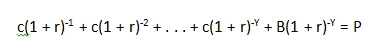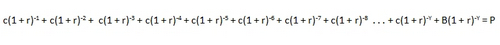# Calculate Yield To Maturity For Bonds

There are many different ways to measure the returns of investments. For bondholders, one important consideration when making a intelligent bond investment is the Yield to Maturity for the bond.

The definition of yield to maturity, or YTM, is the return the investor would receive, including all interest payments, if the bond where to be held until it matures and was redeemed at that time. In other words, YTM is the return on a bond held until maturity.

Ironically, one of the frequently asked questions regarding YTM is how to calculate Yield to Maturity manually or what the YTM formula is so that it can be figured out by hand.

Let me just help you out here. You don't calculate YTM by hand. You don't use the formula, and you don't need to know it, even if you are a math major or a MBA student.

Right about now, you might be thinking, well what did they do to calculate bond yield and YTM before computers and financial calculators could do the YTM math?

The answer is that they had bond tables. These tables were big tables like those you see in the back of an atlas that show you how many miles it is between two cities. You find Miami in one column and then find Chicago in one row and then where the two intersect, that number is how many miles it is to Chicago from Miami. They had the same kind of tables for Yield to Maturity, and other bond yield calculations. It simply was not worth anyone's time to do the math.

Calculating a bond's yield is not hard, in that the math involved is not that complicated. However, what makes it so time consuming and utterly not worth the time is that there are additional terms for each time period. In other words, if there are 35 coupon payments left until your bond matures, your YTM formula will have over 35 terms to calculate.

Here is the basic formula:Here is what it looks like when you START to do the math:I think you start to get the picture. If you have 10 years left on a bond that pays semi-annually, you'll have 20 of those little terms to figure out in the middle of the formula. Not only is that time consuming, it's error prone as well.

Just use one of the tons of calculators out there. Try this one at Morningstar if you want one that is really simple.